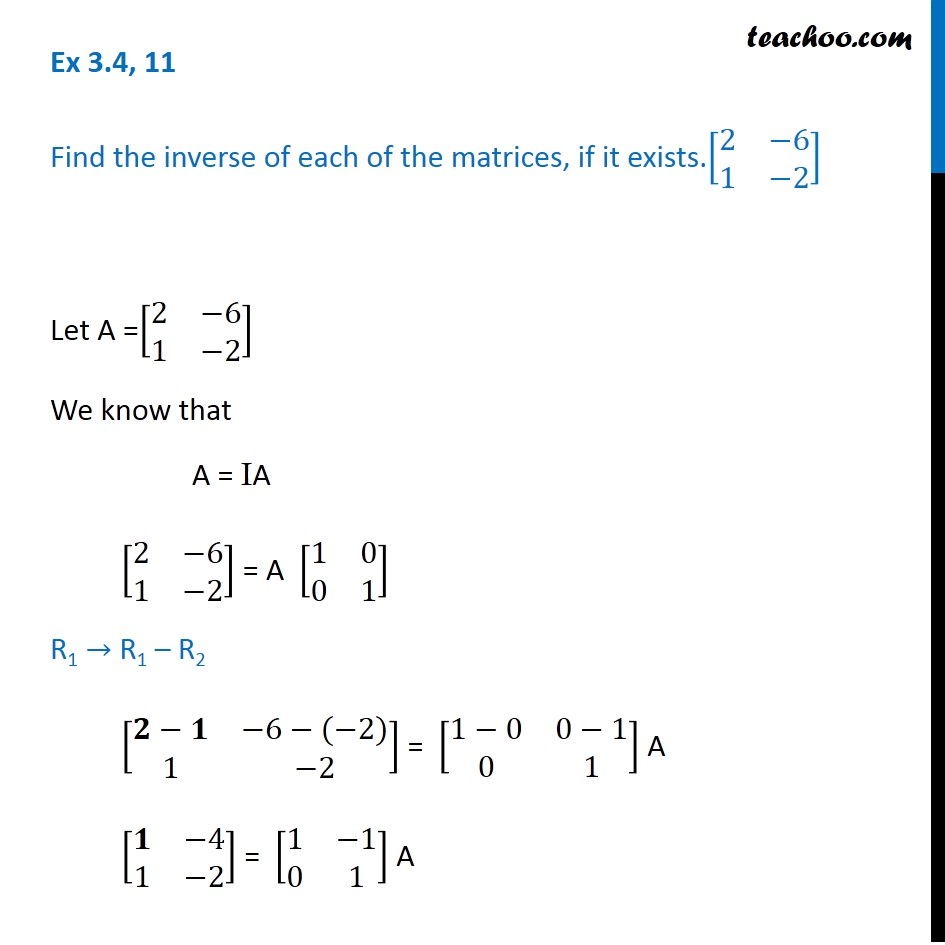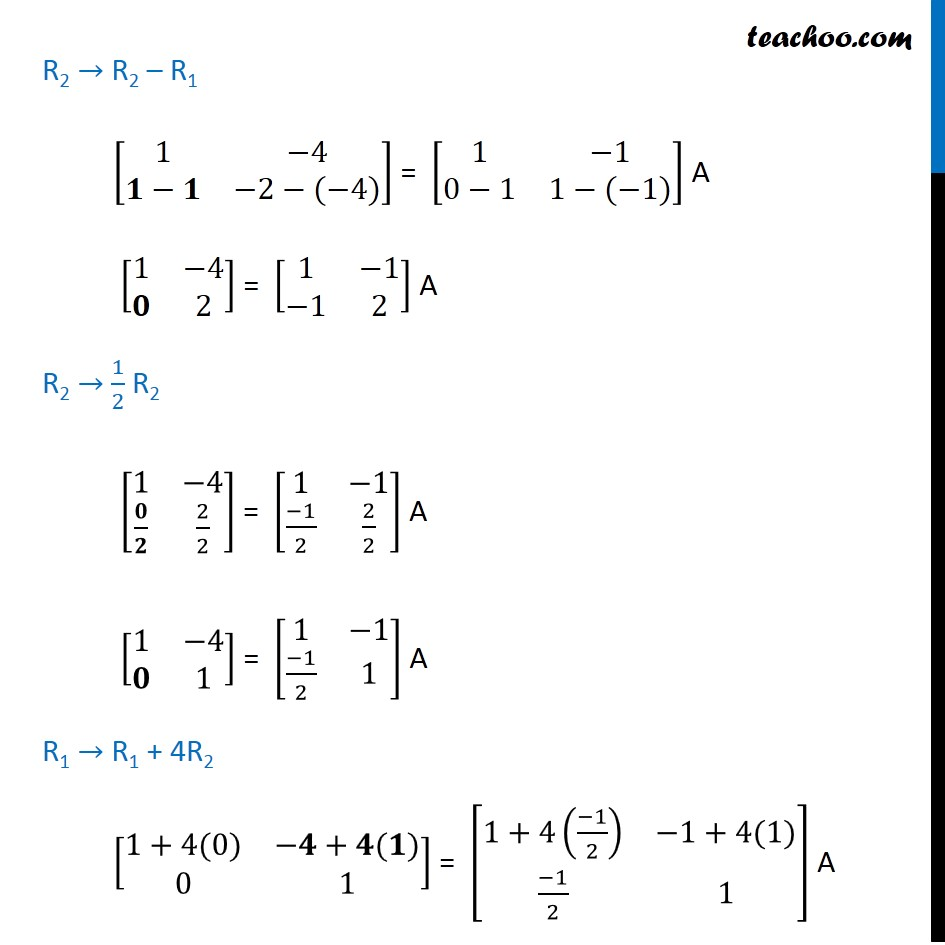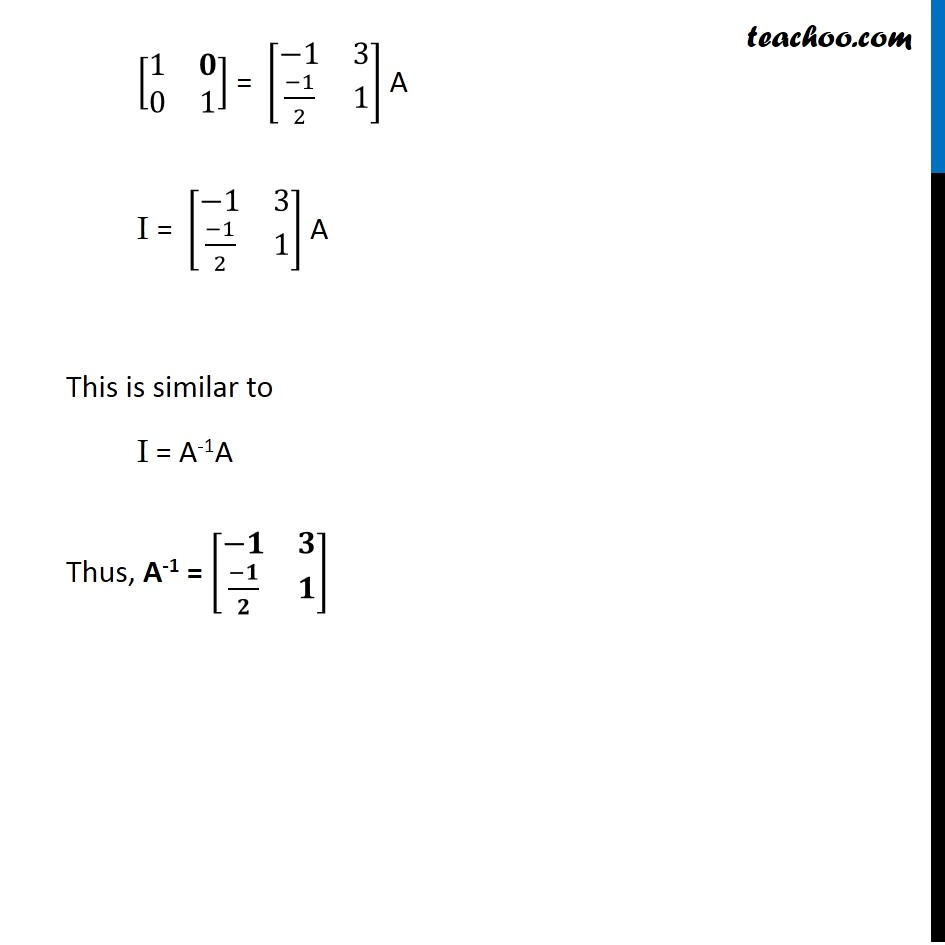Ex 3.4

Chapter 3 Class 12 Matrices
Serial order wiseLearn in your speed, with individual attention - Teachoo Maths 1-on-1 Class

### Transcript

Ex 3.4, 11 Find the inverse of each of the matrices, if it exists.[■8(2&−6@1&−2)] Let A =[■8(2&−6@1&−2)] We know that A = IA [■8(2&−6@1&−2)] = A [■8(1&0@0&1)] R1 → R1 – R2 [■8(𝟐−𝟏&−6−(−2)@1&−2)] = [■8(1−0&0−1@0&1)] A [■8(𝟏&−4@1&−2)] = [■8(1&−1@0&1)] A R2 → R2 – R1 [■8(1&−4@𝟏−𝟏&−2−(−4))] = [■8(1&−1@0−1&1−(−1))] A [■8(1&−4@𝟎&2)] = [■8(1&−1@−1&2)] A R2 → 1/2 R2 [■8(1&−4@𝟎/𝟐&2/2)] = [■8(1&−1@(−1)/2&2/2)] A [■8(1&−4@𝟎&1)] = [■8(1&−1@(−1)/2&1)] A R1 → R1 + 4R2 [■8(1+4(0)&−𝟒+𝟒(𝟏)@0&1)] = [■8(1+4((−1)/2)&−1+4(1)@(−1)/2&1)] A [■8(1&𝟎@0&1)] = [■8(−1&3@(−1)/2&1)] A I = [■8(−1&3@(−1)/2&1)] A This is similar to I = A-1A Thus, A-1 = [■8(−𝟏&𝟑@(−𝟏)/𝟐&𝟏)]